Sunil Ray — Published On April 9, 2015 and Last Modified On July 22nd, 2022

### Introduction

Exploring data sets and developing deep understanding about the data is one of the most important skills every data scientist should possess. People estimate that the time spent on these activities can go as high as 80% of the project time in some cases.

Python has been gaining a lot of ground as preferred tool for data scientists lately, and for the right reasons. Ease of learning, powerful libraries with integration of C/C++, production readiness and integration with web stack are some of the main reasons for this move lately.

In this guide, I will use NumPy, Matplotlib, Seaborn, and Pandas to perform data exploration. These are powerful libraries to perform data exploration in Python. The idea is to create a ready reference for some of the regular operations required frequently. I am using an iPython Notebook to perform data exploration and would recommend the same for its natural fit for exploratory analysis.

In case you missed it, I would suggest you to refer to the baby steps series of Python to understand the basics of python programming.

### Part 1: How to load data file(s) using Pandas?

Input data sets can be in various formats (.XLS, .TXT, .CSV, JSON ). In Python, it is easy to load data from any source, due to its simple syntax and availability of predefined libraries, such as Pandas. Here I will make use of Pandas itself.

Pandas features a number of functions for reading tabular data as a Pandas DataFrame object. Below are the common functions that can be used to read data (including read_csv in Pandas):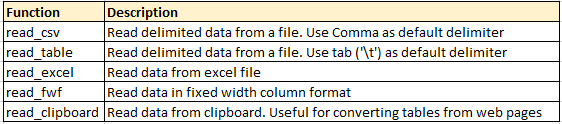Code

```Code
Output
print df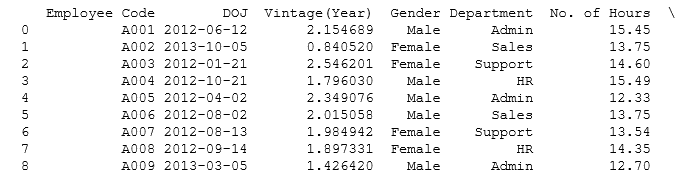```

Code:

`df=pd.read_csv("E:/Test.txt",sep='\t') # Load Data from text file having tab '\t' delimeter print df`

Output

### Part 2: How to convert a variable to a different data type?

Converting a variable data type to others is an important and common procedure we perform after loading data. Let’s look at some of the commands to perform these conversions:

• #### Convert numeric variables to string variables and vice versa

```srting_outcome = str(numeric_input) #Converts numeric_input to string_outcome
integer_outcome = int(string_input) #Converts string_input to integer_outcome
float_outcome = float(string_input) #Converts string_input to integer_outcome```

The later operations are especially useful when you input value from user using raw_input(). By default, the values are read at string.

• #### Convert character date to Date:

There are multiple ways to do this. The simplest would be to use the datetime library and strptime function. Here is the code:

```from datetime import datetime
char_date = 'Apr 1 2015 1:20 PM' #creating example character date
date_obj = datetime.strptime(char_date, '%b %d %Y %I:%M%p')
print date_obj```

### Part 3: How to transpose a Data set or dataframe using Pandas?

Here, I want to transpose Table A into Table B on the variable Product. This task can be accomplished by using Pandas dataframe.pivot: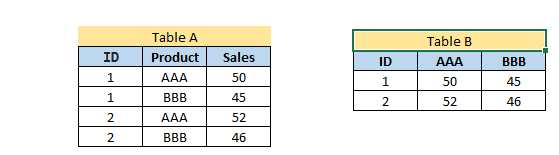Code

#Transposing Pandas dataframe by a variable

```df=pd.read_excel("E:/transpose.xlsx", "Sheet1") # Load Data sheet of excel file EMP
print df
result= df.pivot(index= 'ID', columns='Product', values='Sales')
result
```

Output

### Part 4: How to sort a Pandas DataFrame?

Sorting of data can be done using dataframe.sort(). It can be based on multiple variables and ascending or descending both orders.

Code

```#Sorting Pandas Dataframe
print df.sort(['Product','Sales'], ascending=[True, False])```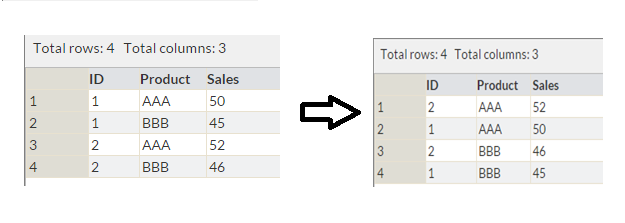Above, we have a table with variables ID, Product and Sales. Now, we want to sort it by Product and Sales (in descending order) as shown in table 2.

### Part 5: How to create plots (Histogram, Scatter, Box Plot)?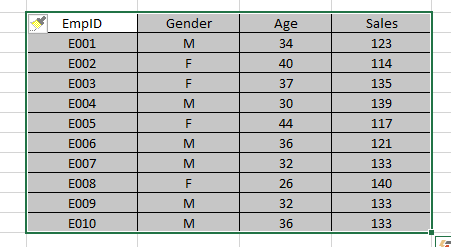Data visualization always helps to understand the data easily. Python has libraries like matplotlib and seaborn to create multiple graphs effectively. Let’s look at the some of the visualizations to understand below behavior of variable(s) .

• The distribution of age
• Relation between age and sales; and
• If sales are normally distributed or not?

#### Histogram:

Code

`#Plot Histogram`
```import matplotlib.pyplot as plt
import pandas as pd```
`df=pd.read_excel("E:/First.xlsx", "Sheet1")`
```#Plots in matplotlib reside within a figure object, use plt.figure to create new figure
fig=plt.figure()```
```#Create one or more subplots using add_subplot, because you can't create blank figure
```#Variable
ax.hist(df['Age'],bins = 5)```
```#Labels and Tit
plt.title('Age distribution')
plt.xlabel('Age')
plt.ylabel('#Employee')
plt.show()
```

Output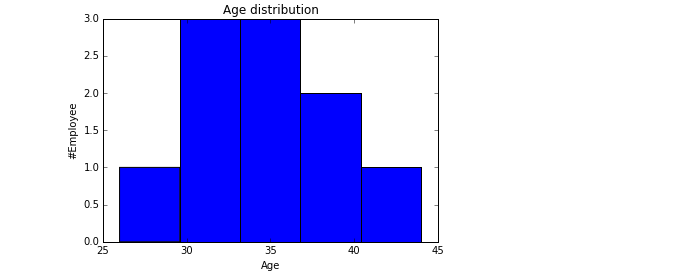#### Scatter plot:

Code

`#Plots in matplotlib reside within a figure object, use plt.figure to create new figure fig=plt.figure()`
```#Create one or more subplots using add_subplot, because you can't create blank figure
```#Variable
ax.scatter(df['Age'],df['Sales'])```
```#Labels and Tit
plt.title('Sales and Age distribution')
plt.xlabel('Age')
plt.ylabel('Sales')
plt.show()
```

Output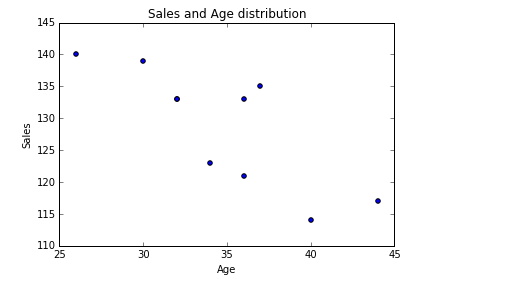#### Box-plot:

Code

```import seaborn as sns
sns.boxplot(df['Age'])
sns.despine()
```

Output

### Part 6: How to generate frequency tables with Pandas?

Frequency Tables can be used to understand the distribution of a categorical variable or n categorical variables using frequency tables.

Code

`import pandas as pd`
```df=pd.read_excel("E:/First.xlsx", "Sheet1")
print df```
```test= df.groupby(['Gender','BMI'])
test.size()
```

Output

### Part 7: How to do sample Data set in Python?

To select sample of a data set, we will use library numpy and random. Sampling of data set always helps to understand data quickly.

Let’s say, from EMP table, I want to select random sample of 5 employees.

Code

`#Create Sample dataframe`
```import numpy as np
import pandas as pd
from random import sample```
```# create random index
rindex = np.array(sample(xrange(len(df)), 5))```
```# get 5 random rows from the dataframe df
dfr = df.ix[rindex]
print dfr
```

Output

### Part 8: How to remove duplicate values of a variable in a Pandas Dataframe?

Often, we encounter duplicate observations. To tackle this in Python, we can use dataframe.drop_duplicates().

Code

`#Remove Duplicate Values based on values of variables "Gender" and "BMI"`
```rem_dup=df.drop_duplicates(['Gender', 'BMI'])
print rem_dup
```

Output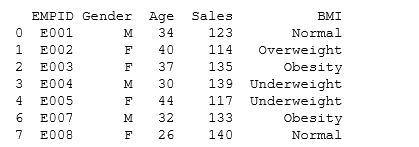### Part 9: How to group variables in Pandas to calculate count, average, sum?

To understand the count, average and sum of variable, I would suggest you use dataframe.describe() with Pandas groupby().

Let’s look at the code:

Code

```test= df.groupby(['Gender'])
test.describe()

```

Output

### Part 10: How to recognize and Treat missing values and outliers in Pandas?

To identify missing values , we can use dataframe.isnull(). You can also refer article “Data Munging in Python (using Pandas)“, here we have done a case study to recognize and treat missing and outlier values.

Code

```# Identify missing values of dataframe
df.isnull()

```

Output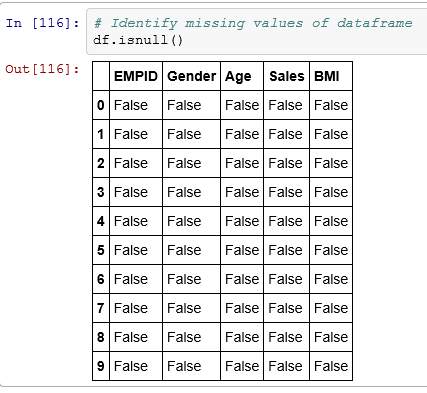To treat missing values, there are various imputation methods available. You can refer these articles for methods to detect Outlier and Missing values. Imputation methods for both missing and outlier values are almost similar. Here we will discuss general case imputation methods to replace missing values. Let’s do it using an example:

Code:

```#Example to impute missing values in Age by the mean
import numpy as np
meanAge = np.mean(df.Age)     #Using numpy mean function to calculate the mean value
df.Age = df.Age.fillna(meanAge) #replacing missing values in the DataFrame```

### Part 11: How to merge / join data sets and Pandas dataframes?

Joining / merging is one of the common operation required to integrate datasets from different sources. They can be handled effectively in Pandas using merge function:

Code:

```df_new = pd.merge(df1, df2, how = 'inner', left_index = True, right_index = True) # merges df1 and df2 on index
# By changing how = 'outer', you can do outer join.
# Similarly how = 'left' will do a left join
# You can also specify the columns to join instead of indexes, which are used by default.```

### End Notes:

In this comprehensive guide, we looked at the Python codes for various steps in data exploration and munging. We also looked at the python libraries like Pandas, Numpy, Matplotlib and Seaborn to perform these steps. In next article, I will reveal the codes to perform these steps in R.

Also See: If you have any doubts pertaining to Python, feel free to discuss with us.

### If you like what you just read & want to continue your analytics learning, subscribe to our emails, follow us on twitter or like our facebook page.###### Sunil Ray

I am a Business Analytics and Intelligence professional with deep experience in the Indian Insurance industry. I have worked for various multi-national Insurance companies in last 7 years.

## 14 thoughts on "Ultimate guide for Data Exploration in Python using NumPy, Matplotlib and Pandas"###### Vikas says:April 10, 2015 at 6:25 am
Need the same thing/article in R. Please do need full asap. Thanks. Reply###### Sandeep Karkhanis says:April 10, 2015 at 9:45 am
About data imputation... I'm struggling to find methods (read packages / libraries) to carry out imputation in Python beyond the usual mean/ median approach...coming from an R background , there are tonnes of good packages to carry out very sophisticated imputation...this is something I miss in Python (it may be my lack of knowledge). i tried r2py to call imputation packages from R in python but it doesnt run as smoothly do you have suggestions in this regards? Reply###### Ken Stulce says:April 16, 2015 at 12:10 pm
Fantastic article with great examples! Thank you. Reply###### Sunil Ray says:April 27, 2015 at 5:51 am
Hi Vikas, Guide for Data Exploration using R is live now. You can access it here http://www.analyticsvidhya.com/blog/2015/04/comprehensive-guide-data-exploration-r/ Thanks Reply###### brianpiercy says:June 05, 2015 at 5:31 pm
Awesome list. Thanks so much! Reply###### subbrao says:August 27, 2015 at 4:54 am
Great article. I learned something today. Any books which helps me to do these kind of data analysis? Reply###### K CHENDRA TEJA says:August 27, 2015 at 5:49 pm###### venugopal says:November 30, 2015 at 12:01 pm###### Animesh says:December 22, 2016 at 8:08 am###### Sunny says:February 27, 2017 at 7:05 am